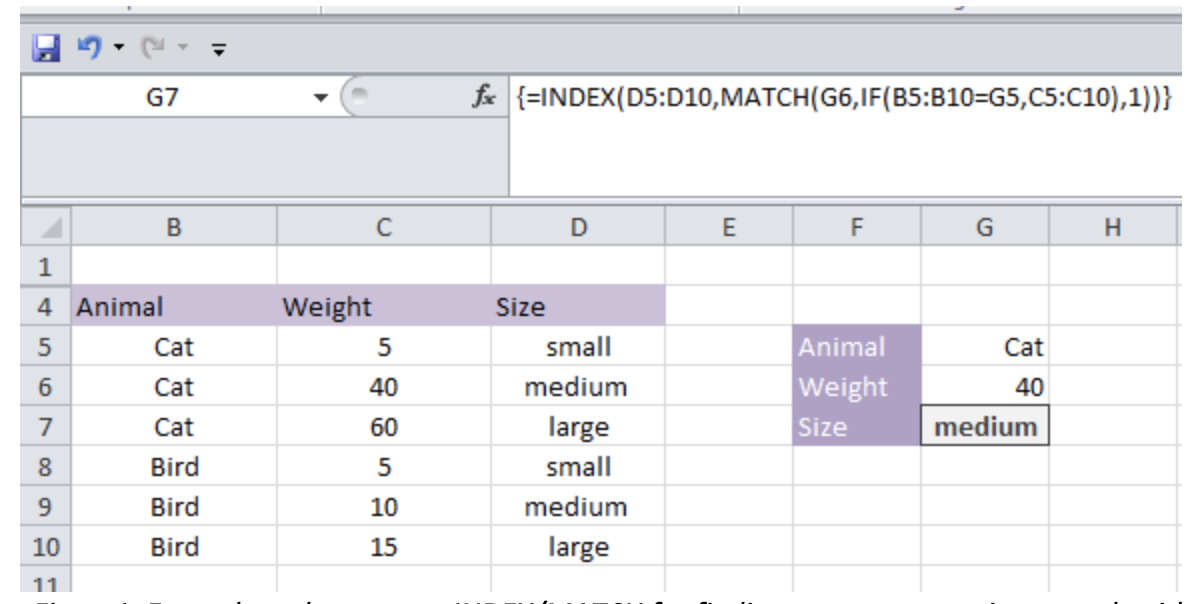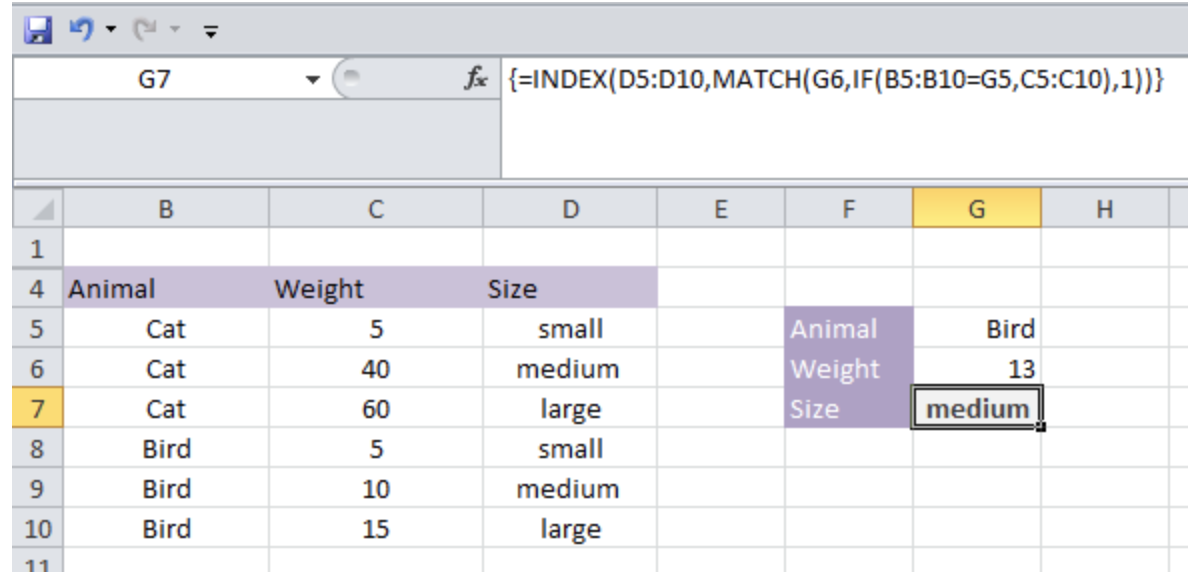Get instant live expert help with Excel or Google Sheets“My Excelchat expert helped me in less than 20 minutes, saving me what would have been 5 hours of work!”

#### Post your problem and you’ll get Expert help in seconds.

Your message must be at least 40 characters
Our professional Expert are available now. Your privacy is guaranteed.

# How to Find Approximate Match with Multiple Criteria in Excel

Microsoft Excel features a series of complicated formula and equations, which can implement for simplifying a problem. It also features INDEX and MATCH functions for Lookup & Reference purposes. When these two functions are combined together logically, it acts in combination to find an approximate match with multiple criteria.  Let’s have a look at the following guide for better clarification:

## Finding Approximate Match with Multiple Criteria in Excel:Figure1. Example of how to use INDEX/MATCH for finding out an approximate match with multiple criteria

### Formula:

`=INDEX(D5:D10,MATCH(G7,IF(B5:B10=G6,C5:C10),1))`

### Explanation of formula:

In certain cases, an Excel user is often required to find out an approximate match with respect to multiple criteria. It requires the user to specify the stipulation of the items. For example, let’s have a look at the screenshot attached above; a user is required to specify the size of the cat, and it is given a range of weight options in the array. Using the formula highlighted above as well as in Excel’s formula bar, it will be able to return value to the selected cell (G7).

The main objective of using the formula is based on looking up and approximates a corresponding match on the basis of its given criteria. A user can use array formula using MATCH and INDEX; however, it should also integrate IF function into the formula for specifying its conditional format.

### How does the formula work?

The equation is a combination of INDEX and MATCH, which is further supplemented by a conditional IF function. The addition of {} to the equation converts it into an array formula, which should be maneuvered using CSE (Control +Shift+ Enter).

Also, once you have entered the formula, you can manually change its values, and it would return the values accordingly. Let’s have a look at the following example:Figure2. Example of changing the specifications to find out the approximate match with multiple criteria

Still need some help with Excel formatting or have other questions about Excel? Connect with a live Excel expert here for some 1 on 1 help. Your first session is always free.

### Did this post not answer your question? Get a solution from connecting with the expert.Another blog reader asked this question today on Excelchat:
Solution examplesI have a file in which I need to somehow use a conditional formatting for the same names of the data available, or if there is any other way to calculate the frequency of one particular name appearing.
Solved by K. F. in 51 minsHi, I have created a drop down list and in another cell I have used an IF function which is dependent on the drop down list. However, when i change the selected option from the drop down list, the IF function cell does not update accordingly
Solved by C. C. in 17 minsindex and match with duplicates. I need to use another column as a reference, so my return value has two match the value of two things for it to return
Solved by E. H. in 60 minsHow can I automatically change different cells and the information they display if we select different option from a drop down list?
Solved by V. U. in 59 minsI'm looking to offset from =today(), for example: If cell A13=today(), display cell A20. If cell B13=today(), display cell B20. If cell C13=today(), display cell C20. I want to do this for 365 rows. Is there a simpler way of doing this than a huge nested IF statement?
Solved by K. H. in 34 mins## Subscribe to Excelchat.coAnother blog reader asked this question today on Excelchat: